# Maximum Subsequence Sum of an Array

First, let’s define what a maximum subsequence sum problem is. Suppose we have an array [5, -3, 4, -7, 8, 7, 4, -1] then we would want to find a contiguous sequence that has the maximum sum. In this case, subsequence [8, 7, 4] has the maximum sum i.e. 19. It’s worth noting that if the array did not have any negative numbers, the subsequence with maximum sum would be simply the given array. And, for this problem, let’s consider if all elements are negative, the maximum sum would be 0.

Mathematically, if A is an array with ‘n’ elements, we could define the maximum subsequence sum of A as

(1)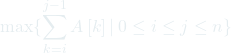Let’s solve this problem in few ways

1. A simple brute force approach to generate all subsequence sums and return the highest sum. Let’s see a code to implement definition (1) in python

def max_subsequence_sum(A):
max_sum = 0
n = len(A)
for i in range(n):
for j in range(n):
curr_sum = 0
for k in range(i, j):
curr_sum += A[k]
max_sum = max(max_sum, curr_sum)
return max_sum


When we run this function with [5, -3, 4, -7, 8, 7, 4, -1], it returns 19 which is a correct answer. If we look into the code, this function has 3 nested loops, and if the size of the array becomes very large, this function takes really long time to get the results. For this function, we have: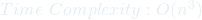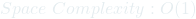So we definitely need to improve the time complexity of this function.

2. Removing unnecessary loops from our previous solution. Let’s see how we can optimize the above function by removing the second for loop. Python code would look something like

def max_subsequence_sum(A):
max_sum = 0
n = len(A)
for i in range(n):
curr_sum = 0
for k in range(i, n):
curr_sum += A[k]
max_sum = max(max_sum, curr_sum)
return max_sum

For this function, we would then have: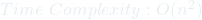Can we do better? Let’s look at a top-down approach.

3. A Top-Down Solution. For a given array A with n number of elements i.e. A[0…n-1], we can represent the maximum subsequence sum of A as sn. And sn-1 would then be A[0…n-2] for n > 0. Then our overall maximum subsequence sum would be the maximum of sn-1 and all of the sums of subsequences A[i…n-1] for 0 <= i <= n which we represent as tn.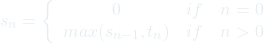And, this sum of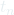, which we call maximum suffix sum will be defined as

(2)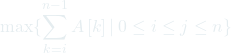And we can calculateas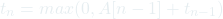So, implementing the given specification as a python code, we would get

def max_subsequence_sum(A):
n = len(A)
if n == 0:
return 0
else:
return max(max_subsequence_sum(A[:n-1]), max_suffix_sum(A[:n]))

def max_suffix_sum(A):
n = len(A)
if n == 0:
return 0
else:
return max(0, A[n-1] + max_suffix_sum(A[:n-1]))


Running max_subsequence_sum with [5, -3, 4, -7, 8, 7, 4, -1] indeed gives us 19. However, analyzing the code, this top-down approach still runs in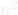time. Even worse, it uses a stack and this stack space grows linearly with n–size of the input. So, for this approach we have,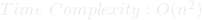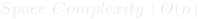Although this approach did not give us an advantage over the previous method, we have techniques to improve the stack usage. However, we can implement this in a bottom-up fashion and remove using recursion altogether. Let’s see how we might do it.

4. Bottom-up approach to calculate maximum subsequence sum. We can optimize the above implementation such that we can compute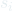immediately after computing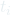. Let’s look at the code how we can implement it.

def max_subsequence_sum(A):
max_sum = 0
max_suf_sum = 0
for i in range(len(A)):
max_suf_sum = max(0, max_suf_sum + A[i])
max_sum = max(max_sum, max_suf_sum)
return max_sum

So, we can see that we have optimized our solution a lot and analyzing this implementation, we find that it runs in linear time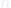with constant space usage. So, for this implementation, we have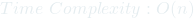What if we also want to return the subsequence as well? This should not be too difficult once we understand the above implementation. Let’s use i_index and j_index as two variables to keep track of our desired subsequence. Then finally we return subsequence with these two indexes as starting and ending index for the maximum subsequence. Let us see in the code how we can implement it.

def max_subsequence_sum(A):
max_sum = 0
max_suf_sum = 0
i_index, j_index = 0, 0
temp_idx = 0

for i in range(len(A)):
max_suf_sum += A[i]
if max_suf_sum > max_sum:
max_sum = max_suf_sum
j_index = i
i_index = temp_idx

if max_suf_sum < 0:
temp_idx = i + 1
max_suf_sum = 0

return A[i_index:j_index+1], max_sum

This implementation still runs in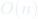with constant space used. Thank you for making it till the end. Yaay!